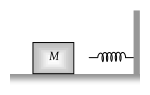The block of mass M moving on the frictionless horizontal surface collides with the spring of spring constant K and compresses it by length L. The maximum momentum of the block after collision is(1) Zero

(2) $\frac{M{L}^{2}}{K}$

(3) $\sqrt{MK}\text{\hspace{0.17em}}L$

(4) $\frac{K{L}^{2}}{2M}$